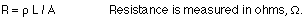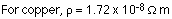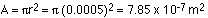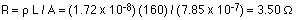## Friday, 3 October 2014

### RESISTANCE AND COST OF ELECTRICAL ENERGY PROBLEMS

1)A copper wire has a length of 160 m and a diameter of 1.00 mm. If the wire is connected to a 1.5-volt battery, how much current flows through the wire?
The current can be found from Ohm's Law, V = IR. The V is the battery voltage, so if R can be determined then the current can be calculated. The first step, then, is to find the resistance of the wire:L is the length, 1.60 m. The resistivity can be found from the table on page 535 in the textbook.The area is the cross-sectional area of the wire. This can be calculated using:The resistance of the wire is then:The current can now be found from Ohm's Law:
I = V / R = 1.5 / 3.5 = 0.428 A
2)One kW-h typically costs about 10 cents, which is really quite cheap. It does add up, though. The following equation gives the total cost of operating something electrical:
Cost = (Power rating in kW) x (number of hours it's running) x (cost per kW-h)
An example...if a 100 W light bulb is on for two hours each day, and energy costs \$0.10 per kW-h, how much does it cost to run the bulb for a month?
Cost = 0.1 kW x 60 hours x \$0.1/kW-h = \$0.6, or 60 cents.
Try this at home - figure out the monthly cost of using a particular appliance you use every day. Possibilities include hair dryers, microwaves, TV's, etc. The power rating of an appliance like a TV is usually written on the back, and if it doesn't give the power it should give the current. Anything you plug into a wall socket runs at 120 V, so if you know that and the current you can figure out how much power it uses.
The cost for power that comes from a wall socket is relatively cheap. On the other hand, the cost of battery power is much higher. \$100 per kW-h, a thousand times more than what it costs for AC power from the wall socket, is a typical value.